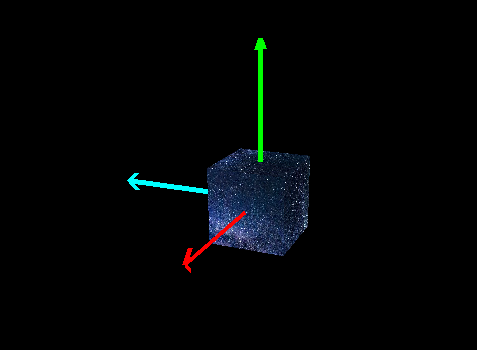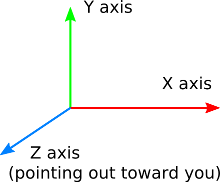# [Rotation of objects using quaternion]

Hello Guys, I hope you’re all doing well.

So I have a scene in which I added a cube and tried to rotate it using a quaternion.
I also attached a coordinate system to it where x is the red axis, y is the green axis and z is the cyan blue axis as shown in the picture below:But I’m wondering, if I use a quaternion to change the orientation of the cube, it rotates with respect to which coordinate system exactly? does it rotate with respect to OpenGL standard coordinate system; I mean the one represented in the picture below:or the coordinate system attached to my cube.

[I applied the rotation using a quaternion then I drew the cube and then I drew its coordinate system]
Thank you All.

I’m not sure what your real reason for using quaternions is, or your real concern about using them (your question is puzzling), but see this: